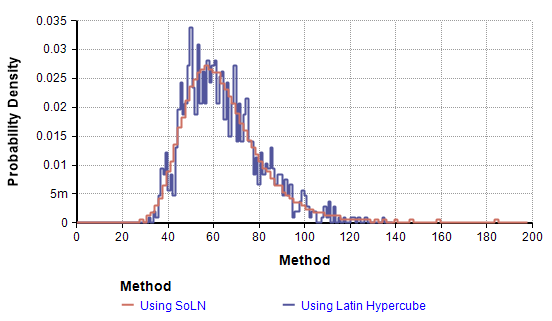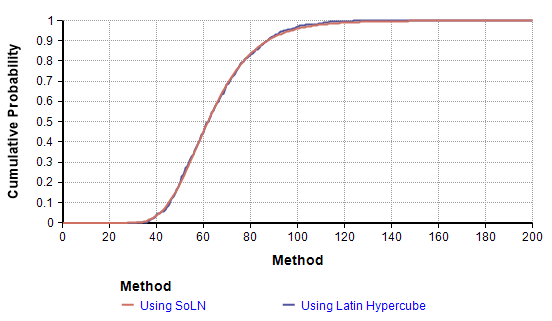# Supporting Materials for Keelin, et. al. (2019)

• Thomas W. Keelin, Lonnie Chrisman, Sam L. Savage (2019), "Extremely accurate sums of Lognormals in closed form using Metalog distributions", submitted to the Proceedings of the 2019 Winter Simulation Conference.

## Abstract

We provide closed-form equations that closely approximate the sum of iid lognormal distributions as a function of lognormal parameters, μ and σ, and of N, the finite number of such distributions to be summed. This is accomplished through a finite table of inputs to a metalog distribution for a limited set of lognormal shape parameters and N’s, which may then be interpolated to estimate the continuous set of lognormal parameters and countable N’s. Uses include estimating total impact of N risk events, each with iid individual lognormal impact, noise in wireless communications networks and other applications. Furthermore, beyond lognormals, the approach may be directly applied to sums of iid variables from virtually any continuous distribution.

## Implementation

Our algorithm computes CDF, Inverse CDF, and probability densities for an Average (or sum) of N Log Normal distributions to a maximum error in CDF of less that 0.01 for all N from 2 to 100 and σ from 0.04 to 1.5. We are providing the following Analytica implementation of the algorithm for download:

We also provide this one that extends the Q-tables (and allowable N) to N=300. Note: The paper only analyzed accuracy up to N=100.

If you want to implement this in a different programming language, we note that it is almost trivial to implement (assuming you have a matrix multiply routine) once you have the Q-tables. See the paper for the details.

The implementations of all these functions is contained within the library and can be freely browsed.

## Q-Tables

The algorithm uses pre-compiled quantiles (the Q-table). The Sum of LogNormal Library.ana includes the Q-table, or you can download just the tables here as an Excel spreadsheet.

## How to use library

If you don't already have Analytica, download and install Analytica Free edition for Windows. Then download the library from the above link. After launching Analytica, start a blank model and select File / Add Module..., and select library file from the link above. If you are unsure, select Embed.

When you have an uncertain variable whose uncertainty is best described as a sum-of-LogNormal distribution, define it using the SoLN distribution function. For example, for a sum of 34 LNs, each with σ=1.13, use:

Chance X1 := SoLN( N:34, sigma:1.13 )

If you are new to Analytica -- drag an oval node from the toolbar to the diagram, title it X1, and press the (x+y) button to edit its definition. For its definition, type SoLN( N:34, sigma:1.13 ). To learn more, see the Analytica Tutorial.

Note: The paper uses the convention of describing a LogNormal by μ and σ, the parameters of the underlying Normal distribution (i.e., so that each component distribution is Exp( Normal( mu, sigma ) ). Note that Analytica's convention is to describe a LogNormal distribution by specifying any 2 statistics of the LogNormal variable itself -- the median, geometric standard deviation, arithmetic mean or arithmetic standard deviation. This library uses the convention of the paper, rather than the standard Analytica convention. The relationship is as follows. If you desire each component distribution to be LogNormal( med, gsdev ), then μ=Ln(med) and σ=Ln(gsdev).

At an infinite simulation sample size, this is equivalent to

Chance X2 := Local n:=1..34 Do LogNormal( 1, Exp(1.13) )

The following graphs compare the histograms of each of these that result from a Latin-Hypercube simulation with a sample size of 1000:The PDF graphs above are histograms of 1000 samples, using a stair-step line style to emphasize the histogram bins. In many cases, Monte Carlo simulation of sums of LogNormals doesn't achieve very good quantile accuracy, even at very large sample sizes, and especially on the tails. Since we're simulating the SoLN here as a single distribution, we get very smooth coverage using Latin Hypercube, compares to more variation in the PDF when 34 independent LogNormals are simulated.

The AoLN(N, sigma, mean) and SoLN(N, sigma, mean) functions act as Analytica distribution functions, running in Monte-Carlo, Latin-Hypercube or Sobol Sampling mode from uncertainty views, and returning the median value in Mid-views.

For analytic calculation of density, cumulative density, or inverse-cumulative density (aka quantiles), the library provides the functions:

• Dens_AoLN( x, N, sigma, mean) and Dens_SoLN( x, N, sigma, mean)
• Cum_AoLN( x, N, sigma, mean) and Cum_SoLN( x, N, sigma, mean)
• Cum_AoLN_Inv( p, N, sigma, mean) and Cum_SoLN_Inv( p, N, sigma, mean)

These use the same naming convention as other analytic distribution functions in Analytica.

## Errata

There is a typo in Equation (4) of the paper, which should have been

$\displaystyle{ Y_{i, k} = \left\{ \begin{array}{cl} 1 & k=1 \\ \ln\left( {{y_i}\over{1-y_i}} \right) & k=2 \\ (y_i - 0.5) \ln\left( {{y_i}\over{1-y_i}} \right) & k=3 \\ y_i - 0.5 & k = 4 \\ (y_i - 0.5)^{{{k-1}\over 2}} & k= 5,7,9 \\ (y_i - 0.5)^{{k\over 2}-1} \ln\left( {{y_i}\over{1-y_i}} \right) & k=6,8 \\ \end{array} \right. }$

where i indexes the y vector and k indexes the basis functions.